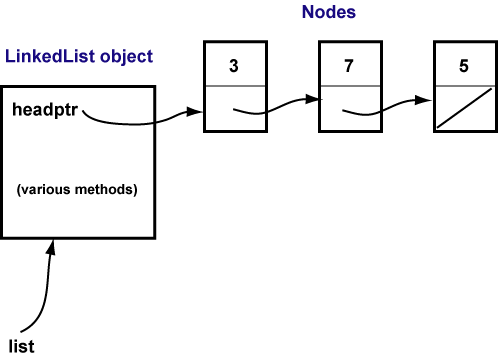Yes.

# Linked List as an Object

In the previous chapter `main()` built a linked list out of `Node` objects, but the linked list itself was not a self-contained object. It would be nice to have an object that consists of the chain of nodes and the methods that manipulate them. This chapter does that.

Here for review is the `Node` class:

```public class Node
{
private Node next;
private int  value;

public Node ( int val )
{
value = val;
next = null;
}

public int  getValue() { return value; }
public Node getNext()  { return next; }

public void setValue( int val ) { value = val; }
public void setNext( Node nxt ) { next = nxt; }

public String toString() { return value + ", "; }
}
```

Here is a sketch of a the `LinkedList` class that contains a pointer to the first node of a chain of nodes:

```public class LinkedList
{

// The constructor creates an empty list
{
}

// Determine if the List is empty
public boolean isEmpty()
{
// future work
}

// Insert one Node containing data at the head
// of the list.  This will be explained in a few pages.
public void insertFirst( int data )
{
Node newFirst = new Node( data );
The goal is to create a `LinkedList` object similar to that pictured:(Thought Question:) Would it be possible to have a `LinkedList` object that does not link to any nodes?